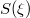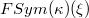﻿

### Centralizers in Hall's universal and direct limits of finitary symmetric groups

#### Abstract

We study the centralizers of finite subgroups in Hall's universal group. We describe the structure of the centralizers of arbitrary finite subgroups in the groups$S(\xi)$ and$FSym(\kappa)(\xi)$ where$S(\xi)$'s are obtained as direct limits of finite symmetric groups and$FSym(\kappa)(\xi)$'s are obtained as direct limits of finitary symmetric groups on the set of infinite cardinality$\kappa$.

DOI Code: 10.1285/i15900932v34n1p105

Keywords: Centralizer; simple locally finite; direct limits of finitary symmetric groups

Full Text: PDF